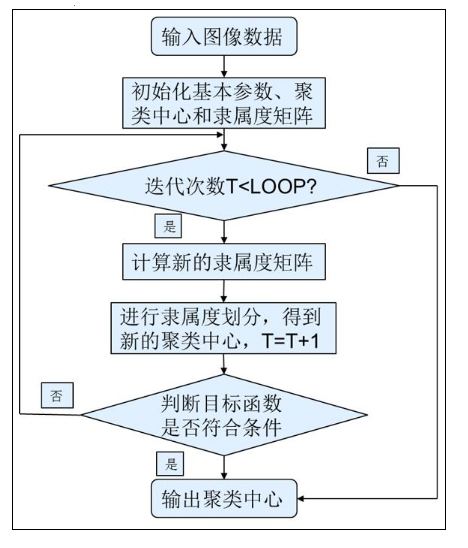• 模糊C均值聚类声纳图像分割：通过模糊C均值聚类的声纳图像分割实现
• Fuzzy C Means - Multi clustering and find center of clusters with Python模糊C均值聚类的python实现，用于实现图像分割图像分割
• 使用matlab中的fcm命令对灰度图像进行模糊c均值聚类的一段小程序，相互交流matlab
• 使用基于模糊 c 均值聚类的机器学习对 2 类和 3 类问题进行一维矩阵分类。 它还包含一个基于矩阵的 AND 门示例和大小为 12 和 3 的输入样本matlab
• 模糊C均值聚类算法可有效的解决遥感信息的不确定性和混合像元的划分。文中基于matlab平台、采用模糊C均值聚类对遥感影像进行分类,并运用混淆矩阵对分类结果进行了精度评定。实验结果表明,基于模糊C均值聚类使得分类...
•研究论文
• 本代码利用MATLAB编写，根据模糊C均值聚类算法（FCM）实现样本点的聚类功能。FCM Matlab
• 使用Opencv实现区域增长法、K均值聚类算法、模糊C均值聚类算法对图像处理；可处理单通道图像以及多通道图像。用法：建立Opencv工程后添加此cpp文件，在该工程中添加lena .jpg图片即可运行程序（当然也可以自己修改...
• 模糊C均值聚类算法代码，实现数据聚类，机器学习代码的一部分。
• 对于彩色图像的模糊c均值聚类，使用c/c++结合opencv2.4.9。
• 模糊C均值聚类简述 By:Yang Liu 1.什么是模糊C均值聚类 模糊c-均值聚类算法 fuzzy c-means algorithm (FCMA)或称（ FCM）。通过优化目标函数得到每个样本点对所有类中心的隶属度，从而决定样本点的类属以达到自动对...
模糊C均值聚类简述
By:Yang Liu
1.什么是模糊C均值聚类
模糊c-均值聚类算法 fuzzy c-means algorithm (FCMA)或称（ FCM）。通过优化目标函数得到每个样本点对所有类中心的隶属度，从而决定样本点的类属以达到自动对样本数据进行分类的目的。无监督机器学习的主要技术之一。
2.模糊C均值聚类涉及的数学方法——拉格朗日乘数法
设给定二元函数z=ƒ(x,y)和附加条件φ(x,y)=0，为寻找z=ƒ(x,y)在附加条件下的极值点，先做拉格朗日函数 $F(x,y,\lambda ) = f(x,y) + \lambda \varphi (x,y)$，其中λ为参数。
令F(x,y,λ)对x和y和λ的一阶偏导数等于零，即
F’(x)=ƒ’x(x,y)+λφ’x(x,y)=0
F’(y)=ƒ’y(x,y)+λφ’y(x,y)=0
F’(λ)=φ(x,y)=0
由上述方程组解出x,y及λ，如此求得的(x,y)，就是函数z=ƒ(x,y)在附加条件φ(x,y)=0下的可能极值点。
若这样的点只有一个，由实际问题可直接确定此即所求的点。
3.模糊C均值聚类的过程简述
隶属度函数是表示一个对象x隶属于集合A的程度的函数，其自变量范围是所有可能属于集合A的对象，取值范围是[0,1]。用$u$表示。
模糊C均值聚类，需要两个参数一个是聚类数目，另一个是一个控制算法的柔性的参数。
算法的输出是聚类中心点向量和一个模糊划分矩阵，这个矩阵表示的是每个样本点属于每个类的隶属度。根据这个划分矩阵按照模糊集合中的最大隶属原则就能够确定每个样本点归为哪个类，聚类中心表示的是每个类的平均特征，可以认为是这个类的代表点。
步骤1：用值在0，1间的随机数初始化隶属矩阵U，使其满足约束条件
步骤2：计算c个聚类中心ci，i=1,…,c。
步骤3：计算价值函数。如果它小于某个确定的阀值，或它相对上次价值函数值的改变量小于某个阀值，则算法停止。
步骤4：计算新的U矩阵。返回步骤2。4.数学推导过程
此时要求的就是价值函数的最小值；进而变成求在隶属度函数的和为1的条件下，价值函数最小的条件极值问题。
价值函数J:$J = \sum\limits_{i = 1}^c {\sum\limits_{j = 1}^n {u_{ij}^m{d_{ij}}} }$ 其中c表示分成了几簇，n表示n个样本，d表示样本到对应样本中心的距离，u表示隶属度函数。
条件极值的条件是:${u_i} = 1$。
构造拉格朗日函数:$J = \sum\limits_{i = 1}^c {\sum\limits_{j = 1}^n {u_{ij}^m{d_{ij}}} } + \sum\limits_{j = 1}^n {{\lambda _j}(\sum\limits_{i = 1}^c {{u_{ij}} - 1} )}$
对变量u和c求导；
对c求导有：$\frac{{\partial J}}{{\partial {c_k}}} = \sum\limits_{i = 1}^c {\sum\limits_{j = 1}^n {\frac{{\partial u_{ij}^m{d_{ij}}}}{{\partial {c_k}}} - \frac{{\partial \sum\limits_{j = 1}^n {{\lambda _j}(\sum\limits_{i = 1}^c {{u_{ij}} - 1} )} }}{{\partial {c_k}}}} }$
其中$\frac{{\partial \sum\limits_{j = 1}^n {{\lambda _j}(\sum\limits_{i = 1}^c {{u_{ij}} - 1} )} }}{{\partial {c_k}}} = 0$
则有$\frac{{\partial J}}{{\partial {c_k}}} = \sum\limits_{i = 1}^c {\sum\limits_{j = 1}^n {\frac{{\partial u_{ij}^m{d_{ij}}}}{{\partial {c_k}}} = } } \sum\limits_{i = 1}^c {\sum\limits_{j = 1}^n {u_{ij}^m\frac{{\partial {d_{ij}}}}{{\partial {c_k}}} = } } u_{kj}^m\sum\limits_{j = 1}^n {\frac{{\partial {d_{ij}}}}{{\partial {c_k}}} = } 0$
由于d是c和x的距离，且倒数最后为0，则非零的系数可以去掉。
最后变成关于c和x的等式，有$\sum\limits_{j = 1}^n {u_k^m{c_k}} - \sum\limits_{j = 1}^n {u_k^m{x_j}} = 0$,
最终得到${c_k} = \frac{{\sum\limits_{j = 1}^n {u_{kj}^m{x_j}} }}{{\sum\limits_{j = 1}^n {u_{kj}^m} }}$。
对隶属函数u进行求导：$\frac{{\partial J}}{{\partial {u_{ij}}}} = \sum\limits_{j = 1}^n {\sum\limits_{i = 1}^c {mu_{ij}^{m - 1}{d_{ij}} + \sum\limits_{j = 1}^n {c{\lambda _j}} } } = \sum\limits_{j = 1}^n {(\sum\limits_{i = 1}^c {mu_{ij}^{m - 1}{d_{ij}}} + c{\lambda _j}) = 0}$
有$\sum\limits_{i = 1}^c {mu_{ij}^{m - 1}{d_{ij}}} + c{\lambda _j} = \sum\limits_{i = 1}^c {(mu_{ij}^{m - 1}{d_{ij}}} + {\lambda _j}) = 0$因为上式中括号函数大于等于零，
所以$mu_{ij}^{m - 1}{d_{ij}} + {\lambda _j} = 0$
得${u_{ij}} = {(\frac{{ - {\lambda _j}}}{{m{d_{ij}}}})^{\frac{1}{{m - 1}}}}$
由于$\sum\limits_{k = 1}^c {{u_{kj}} = 1}$
可得$\sum\limits_{k = 1}^c {{{(\frac{{ - {\lambda _j}}}{{m{d_{kj}}}})}^{\frac{1}{{m - 1}}}}} = 1$
进而有${(\frac{{ - {\lambda _i}}}{m})^{\frac{1}{{m - 1}}}} = \frac{1}{{\sum\limits_{k = 1}^c {{{(\frac{1}{{{d_{kj}}}})}^{\frac{1}{{m - 1}}}}} }}$
最终可得${u_{ij}} = \frac{1}{{\sum\limits_{k = 1}^c {{{(\frac{{{d_{ij}}}}{{{d_{kj}}}})}^{\frac{1}{{m - 1}}}}} }}$.
参考文献：
（1）https://wenku.baidu.com/view/ee968c00eff9aef8941e06a2.html
（2）https://blog.csdn.net/shaoyu_hlq/article/details/56488204
（3）https://www.cnblogs.com/wxl845235800/p/11046248.html


展开全文算法 机器学习
•研究论文
• 论文研究-广义均衡模糊C均值聚类算法.pdf, 模糊C均值聚类(FCM)算法是一种快速有效的聚类算法,但它没有考虑各类样本容量的差异, 其最小化代价函数会导致聚类判决有利于少...
• 求助模糊C均值聚类FCM-searchMub.m 俺现在要用Matlab实现一个 模糊C均值聚类 算法，很着急啊！有谁有的话，请高抬贵手，给俺回一下，谢谢谢谢你啦！！！！matlab
• 求助模糊C均值聚类FCM-readme.txt 俺现在要用Matlab实现一个 模糊C均值聚类 算法，很着急啊！有谁有的话，请高抬贵手，给俺回一下，谢谢谢谢你啦！！！！matlab
• 求助模糊C均值聚类FCM-fcm.m 俺现在要用Matlab实现一个 模糊C均值聚类 算法，很着急啊！有谁有的话，请高抬贵手，给俺回一下，谢谢谢谢你啦！！！！matlab
• 求助模糊C均值聚类FCM-calcStatYm.m 俺现在要用Matlab实现一个 模糊C均值聚类 算法，很着急啊！有谁有的话，请高抬贵手，给俺回一下，谢谢谢谢你啦！！！！matlab
• 求助模糊C均值聚类FCM-calcMub.m 俺现在要用Matlab实现一个 模糊C均值聚类 算法，很着急啊！有谁有的话，请高抬贵手，给俺回一下，谢谢谢谢你啦！！！！matlab
• 针对模糊C均值聚类算法(FCM)存在对初始聚类中心敏感，易陷入局部最优解的不足，将改进的粒子群聚类算法与FCM算法相结合，提出了一种基于粒子群优化的模糊C均值聚类算法。该算法对粒子群初始化空间及粒子移动最大速度...
•MATLAB
•python...